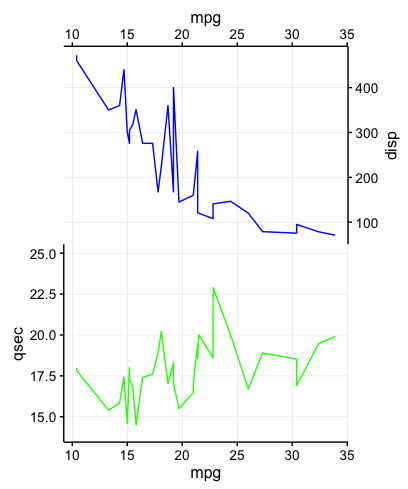The cowplot package defines a function switch_axis_position() that allows us to take the x and/or y axis of a plot and copy or move it to the other side of the plot (y axis to right, x axis to top). Here are some examples of moving or copying various axes. As we can see, this works regardless of the chosen theme.

Importantly, the return object is a gtable, not a ggplot object, so we need to feed it into ggdraw() to display in the usual manner or to save with ggsave() or save_plot().

require(cowplot)
require(grid) # for unit()
theme_set(theme_cowplot(font_size=12)) # reduce default font size
p1 <- ggplot(mtcars, aes(mpg, disp)) + geom_line(colour = "blue")
ggdraw(switch_axis_position(p1 + theme_gray(), axis = 'y'))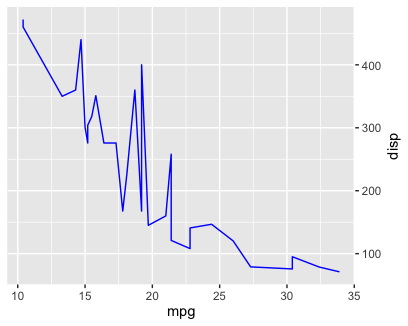ggdraw(switch_axis_position(p1 + theme_bw(), axis = 'x', keep = 'x'))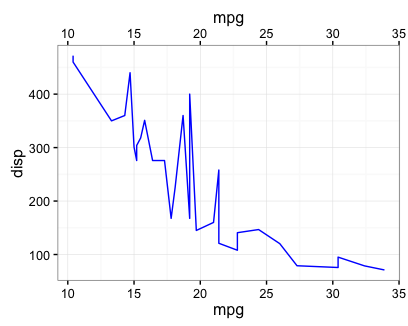ggdraw(switch_axis_position(p1, axis = 'xy', keep = 'xy'))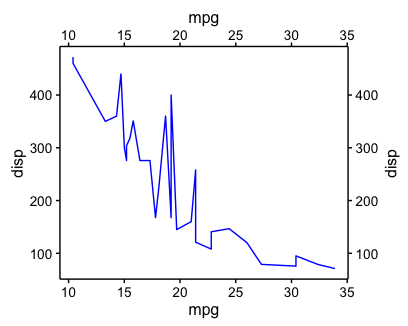ggdraw(switch_axis_position(p1 + theme(axis.ticks.length = unit(0.3, "cm"),
axis.text.x = element_text(margin = margin(0.2, unit = "cm"))), axis = 'xy'))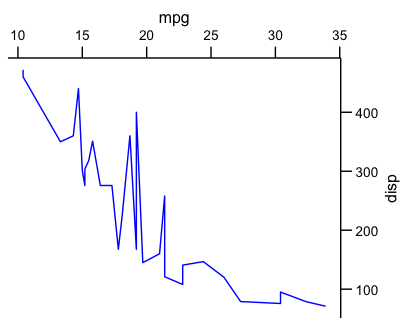Moving the axes also works if there is a legend. What is not currently implemented, however, is moving axes on faceted plots.

p2 <- ggplot(diamonds, aes(clarity, fill = cut)) + geom_bar() +
theme(axis.text.x = element_text(angle = 70, vjust = 0.5))
ggdraw(switch_axis_position(p2, axis = 'x'))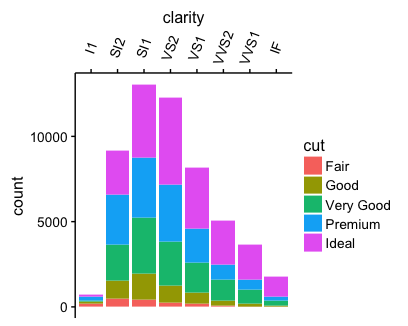When rotating text, you may need to fidle with hjust and vjust to get the labels to be correctly justified.

mtcars2 <- mtcars[1:15, ]
mtcars2name <- row.names(mtcars2) p3 <- ggplot(mtcars2, aes(x = name, y = mpg, fill = name)) + geom_bar(stat = 'identity', position = "identity") + scale_y_reverse() + guides(fill = FALSE) + theme(axis.text.x = element_text(angle = 90, vjust = 0.5, hjust = 1)) ggdraw(switch_axis_position(p3, axis = 'x'))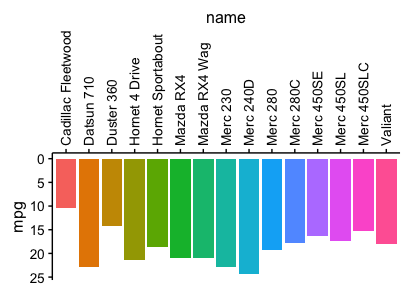In combination with some clever gtable manipulations, we can also align multiple plots with axes that have been switched. Note that the function gtable_squash_rows() I’m using here is one of a few functions cowplot defines for manipulating gtables. require(gtable) # top plot p1 <- ggplot(mtcars, aes(mpg, disp)) + geom_line(colour = 'blue') + background_grid(minor = 'none') g1 <- switch_axis_position(p1, 'xy') # switch both axes g1 <- gtable_squash_rows(g1, length(g1height)) # set bottom row to 0 height

p2 <- ggplot(mtcars, aes(mpg, qsec)) + geom_line(colour = 'green') + ylim(14, 25) +
background_grid(minor = 'none')
g2 <- ggplotGrob(p2)
plot_grid(g1, g2, ncol = 1, align = 'v')